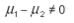## 8.03.2011

### Principles of Two Sample T-test

Two Sample T-Test
This test helps to compare two sample means to discover whether they come from the same population (meaning there is no difference between the two population means). The first step is to obtain the standard error (SE) mean from the sampling distribution of the difference between two sample means. Since the population standard deviations of both of the populations are unknown, the standard error of the two sample means must be estimated in order to fit data to a normalized curve.

How does this works?
The first step to examining a set of data is to establish the specific hypotheses we wish to examine. Specifically, we want to establish a null hypothesis and an alternative hypothesis to be evaluated with data.

As an example for this case:

· Null hypothesis is that the difference between the two groups is 0. Another way of stating the null hypothesis is that the difference between the mean of the treatment group and the mean of the control group is zero.

· Alternative hypothesis - the difference between the observed mean of the treatment group and the expected mean of control group is not zero.

The next step is to determine the best method depending on the sample size. For samples greater or equal than 30, we can use the following method:

Where samples are smaller than 30, we can use the following method:

“Sp” is a pooled estimate of the common population standard deviation. In this method it can be assumed that variances are equal for both populations. If it cannot be assumed, it cannot be used.
The two-sample t-test is illustrated with this example:

 Table 1: Illustration of Two-Sample T-Test N Mean Standard Deviation A Sample 12 92 15 B Sample 15 84 19

Ho is: “The population means are the same, i.e.,Ha is: “The population means are not the same, i.e.,Alpha (or trust level) is to be set at 0.05.

Thus:In the two-sample t-test, the t-statistics are retrieved by subtracting the difference between the two sample means from the null hypothesis, which is:.Looking up t-tables (using spreadsheet software, such as Excel’s TINV function and setting confidence value at 0.05, 25 degrees of freedom), we can find that the critical value of t is 2.06. This means that if the standardized difference between the two samples means (t value above) is larger than 2.06, it can be concluded that there is a significant difference between population means.

Here, 1.19 is less than 2.06; thus the null hypothesis cannot be rejected, that:This T-test application can be used with aircraft engine test cells, whenever there is a hardware or data acquisition method change. This test uses data before and after the required changes and then compare them (using this methodology) to determine if the test cell correlation with the engine is still valid.

Contact TeraSigma Consulting for a more detailed use of this and other statistical tools to support your data analysis services. We can find a tailored solution for your needs.